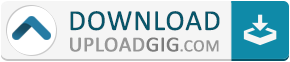#Do not remember me!

### Categories» » » Mathematical Modeling and Computation in Finance With Exercises and Python and MATLAB Computer Codes (True EPUB)

## Mathematical Modeling and Computation in Finance With Exercises and Python and MATLAB Computer Codes (True EPUB)Mathematical Modeling and Computation in Finance With Exercises and Python and MATLAB Computer Codes (True EPUB)
English | 2020 | ISBN: 1786348055 | 1583 pages | True EPUB | 344.99 MB

This book discusses the interplay of stochastics (applied probability theory) and numerical analysis in the field of quantitative finance. The stochastic models, numerical valuation techniques, computational aspects, financial products, and risk management applications presented will enable readers to progress in the challenging field of computational finance.When the behavior of financial market participants changes, the corresponding stochastic mathematical models describing the prices may also change. Financial regulation may play a role in such changes too. The book thus presents several models for stock prices, interest rates as well as foreign-exchange rates, with increasing complexity across the chapters. As is said in the industry, 'do not fall in love with your favorite model.' The book covers equity models before moving to short-rate and other interest rate models. We cast these models for interest rate into the Heath-Jarrow-Morton framework, show relations between the different models, and explain a few interest rate products and their pricing.The chapters are accompanied by exercises. Students can access solutions to selected exercises, while complete solutions are made available to instructors. The MATLAB and Python computer codes used for most tables and figures in the book are made available for both print and e-book users. This book will be useful for people working in the financial industry, for those aiming to work there one day, and for anyone interested in quantitative finance. The topics that are discussed are relevant for MSc and PhD students, academic researchers, and for quants in the financial industry.Name:* E-Mail: Security Code: *